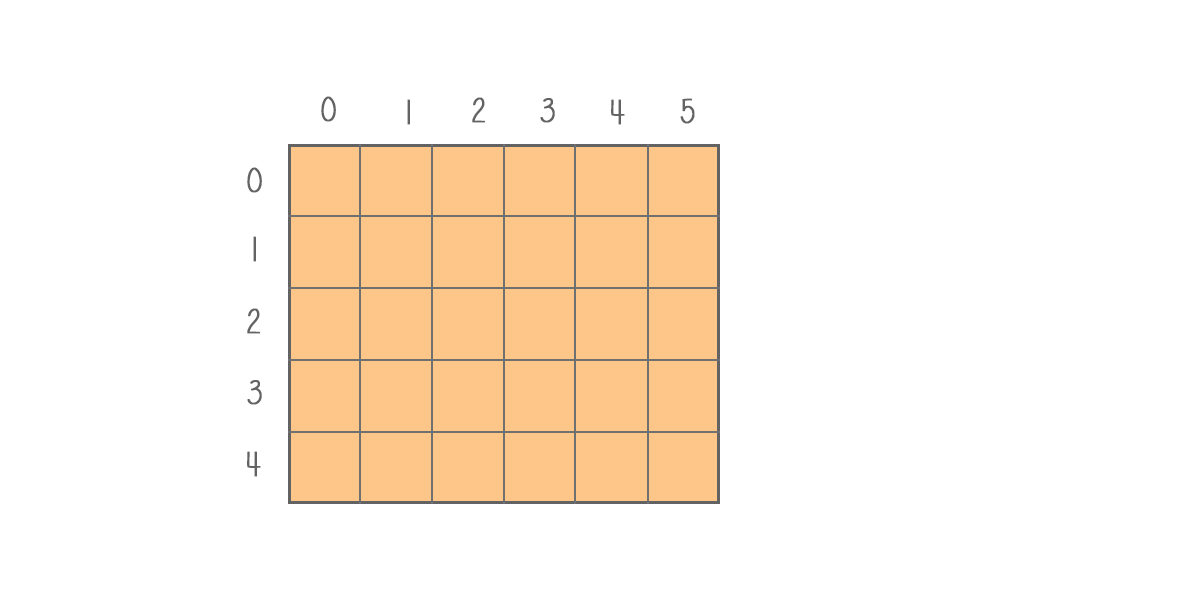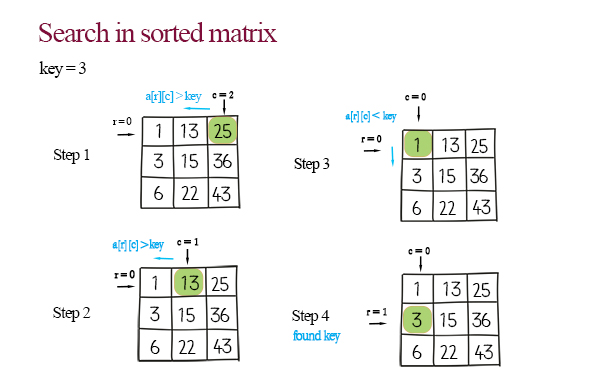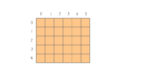A matrix is a two-dimensional or 2d array. The number of rows and the number of columns should be defined when it is declared. The element in the matrix can be assigned and updated by the index of the row and column, as matrix[r][c]. Here is the implementation of matrix operations – initialize, update, search and traversal.### Map of array implementations

Part 1 – array implementation
Part 2 – sorted array implementationPart 3 – matrix 2d array implementation

### Initialize values in matrix

Matrix can be initialized by assigning single value with index of the row and the column. It can also be initialized by coping from another matrix. When copying from another matrix, a nested for loops will be used to iterate through all elements.

## Python

### Update

To update a value is to assign a new value at the index of row and column.

## Python

### Search

There are two ways to search. If the matrix is not sorted, we can scan the elements one by one and rows by rows. If the matrix is sorted horizontally and vertically, we can use two pointers technique to search.

Initialize one pointer pointing to the first row, and the second pointer pointing to the last column. When the element is larger than the key, decrease the column. If the element is less than the key, increase the row. Eventually, the element will be found. This way, the time complexity improves from O(m*n) to O(m+n).

## Doodle### Print

Print is to using two nested loops to print out all elements in the matrix.

## Python